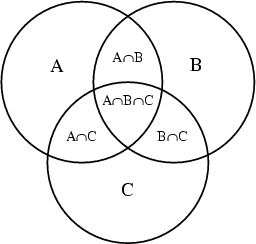# Set Theory - Subset relationship

The subset relationship indicates that every element of a set is also an element of another set.

## Example

A is a subset of B. Every element of A is also an element of B.

$$\Large A subset B$$

Discover MoreDimensional Data Operation - Slice (Or Slicing)

A slice is selecting a subset of a multi-dimensional array. This is equivalent to a filter operation.. Slicing involves using a member of one dimension to provide a slice of the cube. You could...Logical Data Modeling - Transitive Relationship Property

transitive is a relationship property that tells that the relationship follows the following rule: when the relation relates a to b and b to c, then the relation relates a to c. In mathematical notation,...Set Theory - Operator (Operations)

operator in Set Theory that applies to set structure (set, bag) A data operator produce another set. All relational algebra operator are set-operator. A relationship operator produce...Set Theory - Relationship

The relationships between set structure is: given by a mathematical function and is described by the set operators. You can visualize them with venn diagram.Set theory - Equality

in set theory The equality relationship indicates that two sets are equal if they contain exactly the same elements. (There is no order among elements of a set.) Two sets are equal if they contain...